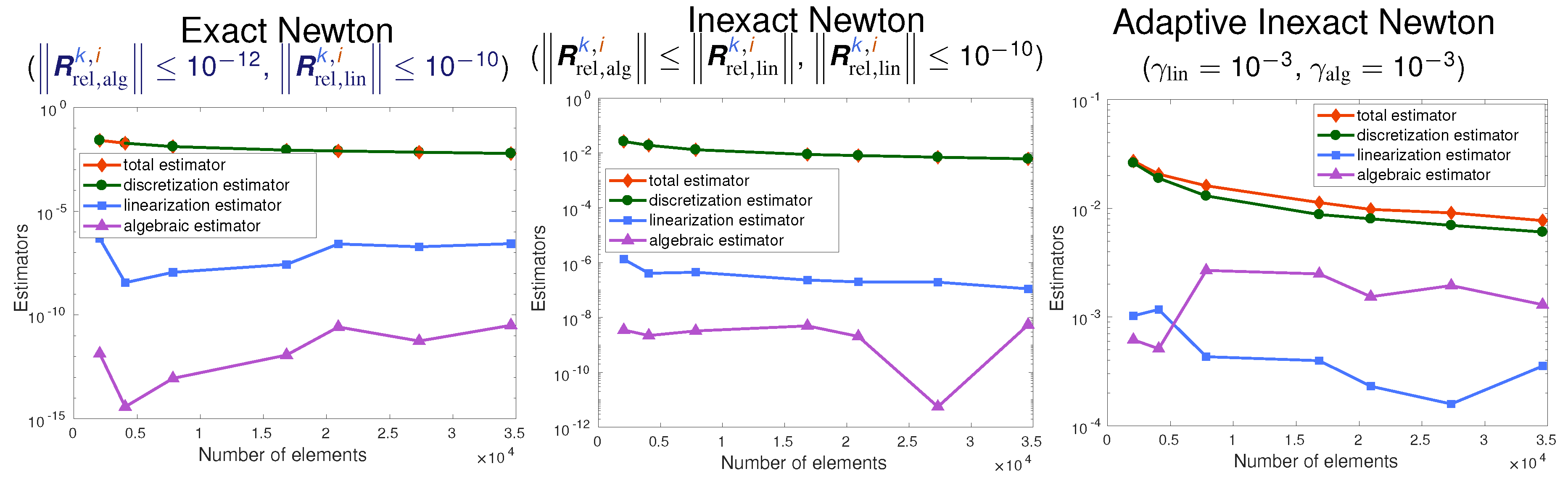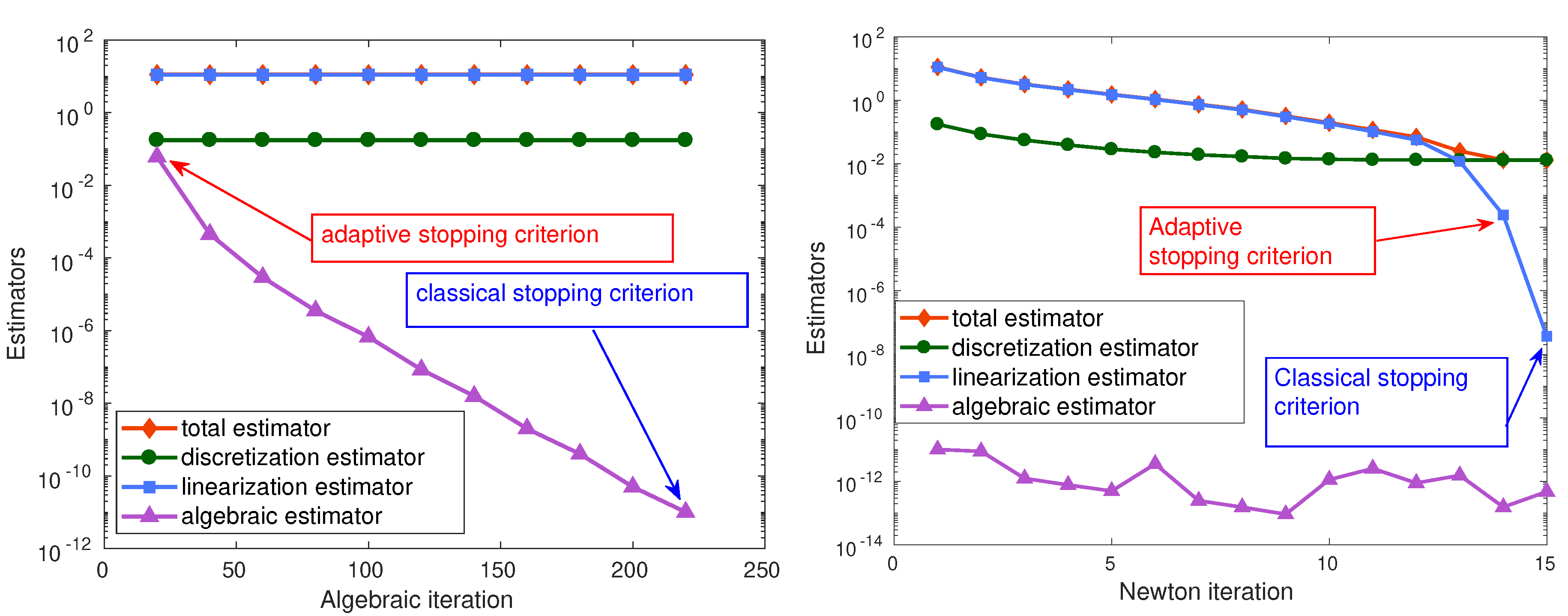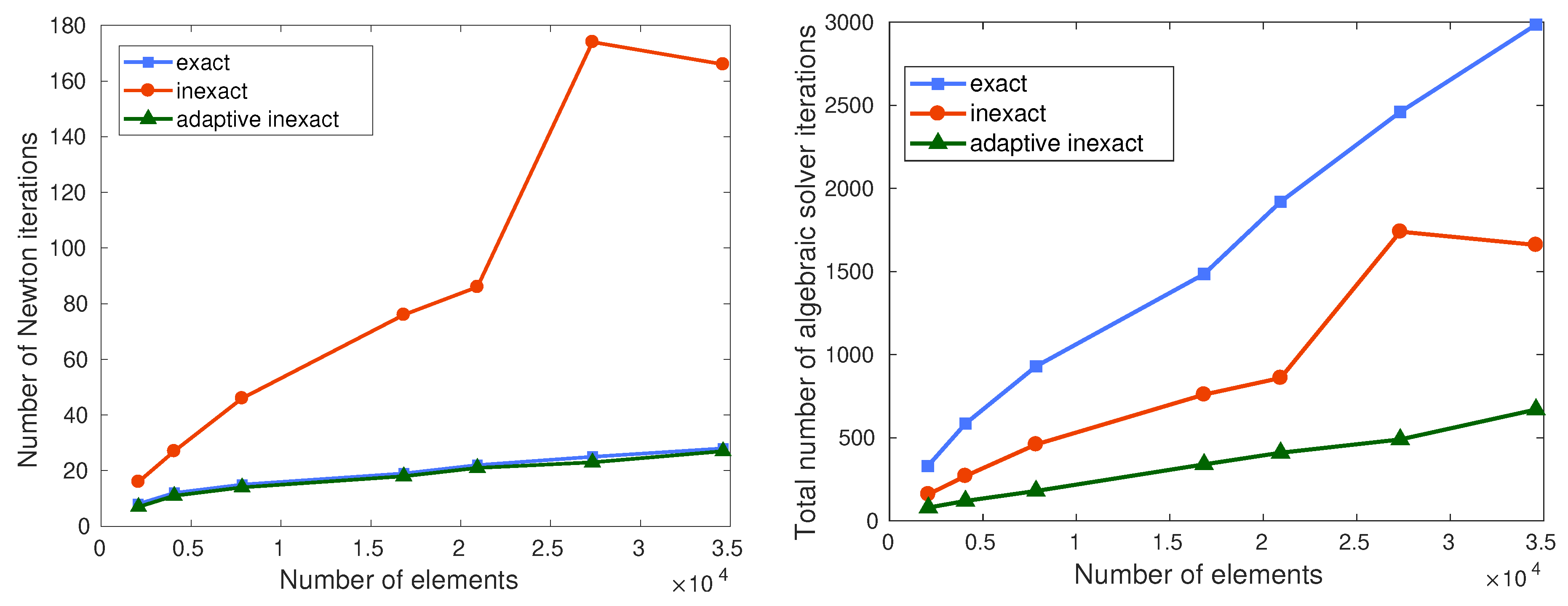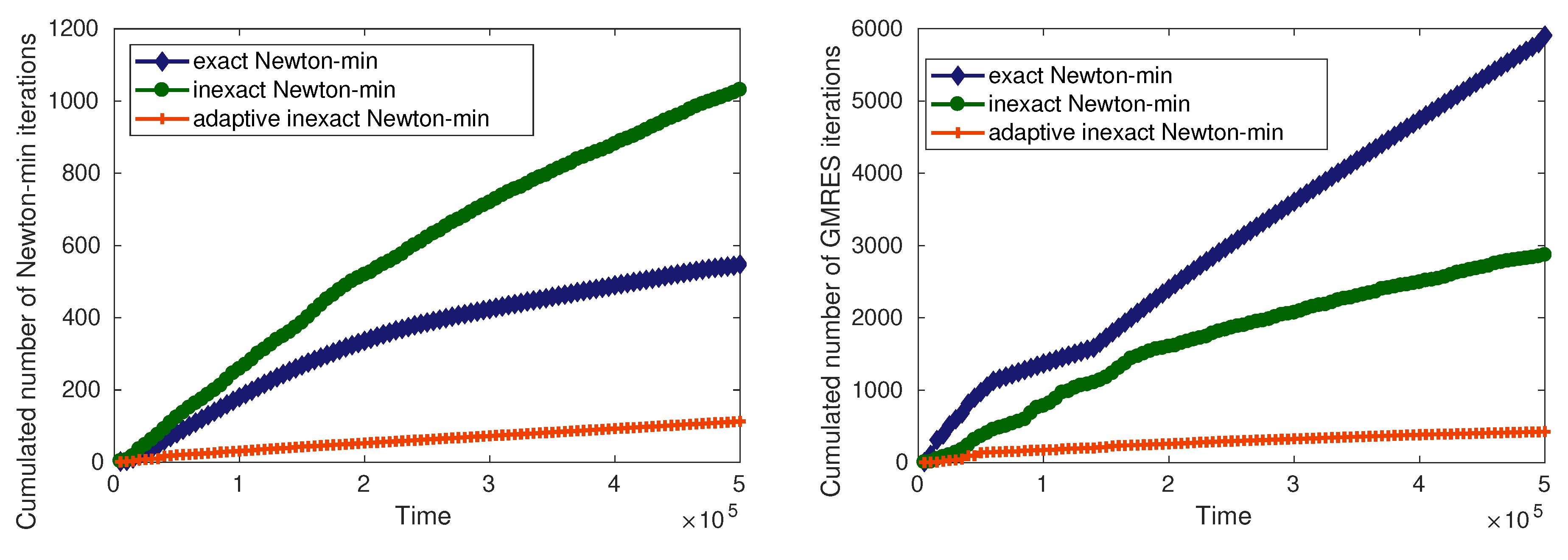# Adaptive inexact semismooth Newton method for variational inequalitiesError components for exact semismooth Newton (left), inexact semismooth Newton (middle), and adaptive inexact semismooth Newton (right) in function of mesh refinement, model steady system of variational inequalitiesA posteriori error estimators in function of algebraic solver iterations (left) and semismooth Newton iterations (right) for the exact/inexact/adaptive inexact semismooth Newton methods, model steady system of variational inequalitiesNumber of semismooth Newton iterations (left) and cumulated number of algebraic solver iterations (right) for the exact/inexact/adaptive inexact semismooth Newton methods, model steady system of variational inequalitiesCumulated number of semismooth Newton iterations (left) and cumulated number of algebraic solver iterations (right) for the exact/inexact/adaptive inexact semismooth Newton methods, unsteady nonlinear system of variational inequalities modeling compositional two-phase flow in porous media with exchange of components between the phases

Main results:

• guaranteed upper bound on the total error;
• distinction of different error components (spatial discretization, temporal discretization, semismooth Newton linearization, algebraic solver);
• design of an adaptive inexact semismooth Newton method for variational inequalities;
• application to unsteady nonlinear coupled degenerate variational inequalities (compositional two-phase flow in porous media with exchange of components between the phases).

Details in Ph.D. thesis of Jad Dabaghi, Ph.D. defense presentation; with Vincent Martin (and Ibtihel Ben Gharbia):

• model steady system of variational inequalities, linear constraints: preprint
• model unsteady system of variational inequalities, linear constraints: preprint
• compositional two-phase flow in porous media, nonlinear constraints: preprint